• 很多人对于四则运算可能不太明天了，下面我们来看看关于php四则运算：中缀表达式转后缀表达式例子，有需要了解的朋友可以一起来看看吧．四则运算表达式，我们书面使用的叫做中缀表达式，而计算器，却更加喜欢后缀...

很多人对于四则运算可能不太明天了，下面我们来看看关于php四则运算：中缀表达式转后缀表达式例子，有需要了解的朋友可以一起来看看吧．
四则运算表达式，我们书面使用的叫做中缀表达式，而计算器，却更加喜欢后缀表达，括号优先级，加减乘除优先级等使得运算中缀四则表达式变得困难。这个时候引入了一种计算机喜欢的格式，叫做后缀表达式。本文以PHP代码，实现中缀表达式转后缀表达式的逻辑。
本文以PHP为代码环境，有人会说高级语言直接写表达式就好了，它们会算，可是他们为什么会算，怎么算的，还是需要把中缀表达式转为后缀表达式。因此本文代码只是模拟一个逻辑。
比如：传统的四则运算表达式(中缀表达式)是9 ( 3 - 1 ) * 3 10 / 2，对应的后缀表达式就是9 3 1 - 3 * 10 2 / 。
转换逻辑：一个字符一个字符的输入，如果是数字则直接输出；如果是左括号则直接入栈；如果是右括号则开始出栈，直到遇到第一次左括号为止；如果是加减乘除，则判断，如果栈顶也是符号，且输入的符号的优先级不高于栈顶的符号优先级，则全部出栈，否则该输入的符号入栈。
/**
* 将输入的字符按照中缀表达式转后缀表达式的规则处理
* @param $str 输入的字符 * @param$stack 栈
* @param $newStrList 新的表达式 */ function suffix($str, &$stack, &$newStrList){
//如果是数字则输出
if(is_numeric($str)){$newStrList .= $str . ' '; } //如果是左括号则入栈 else if($str == '('){
$stack[] =$str;
}
//如果是右括号则将最近的左括号之前的所有数据出栈
else if($str == ')'){ while($arrPop = array_pop($stack)){ if($arrPop == '('){
break;
}
$newStrList .=$arrPop . ' ';
}
}
//如果是加减乘除则判断与栈顶符号优先级
else if(in_array($str, array(' ', '-', '*', '/')) && count($stack) > 0){
$key = (count($stack) - 1);
if(in_array($stack[$key], array(' ', '-', '*', '/'))){
//该符号优先级不高于栈顶符号的
if(checkPriority($str,$stack[$key]) != 1){ for($i=$key;$i>=0; $i--){ if($stack[$i] == '('){ break; }$newStrList .= $stack[$i] . ' ';
unset($stack[$i]);
$stack = array_values($stack);
}
}
}
//本次的符号入栈
$stack[] =$str;
}else{
$stack[] =$str;
}
}
/**
* 判断运算符的优先级
* @param $operatorA * @param$operatorB
* @return A大于B返回1，A等于B返回0，A小于B返回-1
*/
function checkPriority($operatorA,$operatorB){
switch($operatorA){ case ' ': case '-': if($operatorB == ' ' || $operatorB == '-'){ return 0; }else if($operatorB == '*' || $operatorB == '/'){ return -1; } break; case '*': case '/': if($operatorB == ' ' || $operatorB == '-'){ return 1; }else if($operatorB == '*' || $operatorB == '/'){ return 0; } break; default: exit('error'); } } //栈$stack = array();
//待转换的表达式
$strList = '9 ( 3 - 1 ) * 3 10 / 2'; //新的表达式$newStrList = '';
$strList = explode(' ',$strList);
foreach($strList as$str){
if($str != ' '){ suffix($str, $stack,$newStrList);
}
}
//数组反转
while($s = array_pop($stack)){
$newStrList .=$s . ' ';
}
echo $newStrList;  展开全文• 关于后缀表达式的计算机求值以及中缀表达式转后缀表达式的分析请看我的另一篇博客——中缀表达式转前、后缀表达式及其求值后缀表达式求值#include<iostream> #include #include #include<cctype> #include using ... 关于后缀表达式的计算机求值以及中缀表达式转后缀表达式的分析请看我的另一篇博客——中缀表达式转前、后缀表达式及其求值 后缀表达式求值 #include<iostream> #include<iomanip> #include<cstdlib> #include<cctype> #include<cmath> using namespace std; #define MaxSize 100 //堆栈元素的最大个数 #define MaxOP 100 //运算数序列可能的最大长度 #define Number 0 //运算数的标识 #define ZERO 1.0E-10 //ZERO无限接近于0 typedef double ElementType; //将堆栈元素类型具体化 typedef struct SNode *Stack; struct SNode{ ElementType data[MaxSize]; int top; }; //建立空栈 Stack CreateStack() { Stack S = (Stack)malloc(sizeof(struct SNode)); S->top = -1; return S; } //入栈 void Push(Stack PtrS,ElementType X) { if(PtrS->top==MaxSize-1) cout<<"堆栈满"<<endl; else PtrS->data[++(PtrS->top)]=X; } //出栈 #define Error -1 ElementType Pop(Stack PtrS) { if(PtrS->top==-1){ cout<<"空栈"<<endl; return Error; }else{ return (PtrS->data[(PtrS->top)--]); } } int GetOp(char s[]) { /*从输入中获得下一个对象（运算符或运算数），并保存在字符数组s里*/ /*返回值为Number或者运算符本身*/ int i,c; /*跳过表达式前空格等字符*/ while((s = c = getchar()) == ' '||c == '\t'); s = '\0'; if(!isdigit(c)&&c!='.') //如果c是运算符 return c; //直接返回运算符 i = 0; if(isdigit(c)) //如果c是数字，则一直读完连续的数字并存入s while(isdigit(s[++i] = c = getchar())); if(c == '.') //如果有小数点，则一直读完小数点后的连续数字 while(isdigit(s[++i] = getchar())); s[i] = '\0'; //完成对实数字符串的存储 return Number; } //例子 int main() { int Type; ElementType pre; char s[MaxOP]; Stack Ptr = CreateStack(); while((Type = GetOp(s)) != EOF){ //当未读到输入结束时 switch(Type){ case Number:Push(Ptr,atof(s)); break; case'+':Push(Ptr,Pop(Ptr)+Pop(Ptr)); break; case'*':Push(Ptr,Pop(Ptr)*Pop(Ptr)); break; case'-':pre = Pop(Ptr); Push(Ptr,Pop(Ptr)-pre); break; case'/':pre = Pop(Ptr); if(fabs(pre) > ZERO) //检查除法的分母是否为0 Push(Ptr,Pop(Ptr)/pre); else cout<<"error:zero divisor"<<endl; break; case'\n':cout<<setprecision(8)<<Pop(Ptr)<<endl; //小数点后最多显示8位小数(精度为8) break; default:cout<<"error:unknown command "<<s<<endl; break; } } free(Ptr); return 0; } 中缀表达式转后缀表达式 #include<iostream> #include<cstdlib> #include<cctype> using namespace std; #define MaxSize 100 //堆栈元素的最大个数 #define MaxOP 100 //运算数序列可能的最大长度 typedef char ElementType; //将堆栈元素类型具体化 typedef struct SNode *Stack; struct SNode{ ElementType data[MaxSize]; int top; }; //建立空栈 Stack CreateStack() { Stack S = (Stack)malloc(sizeof(struct SNode)); S->top = -1; return S; } //入栈 void Push(Stack PtrS,ElementType X) { if(PtrS->top==MaxSize-1) cout<<"堆栈满"<<endl; else PtrS->data[++(PtrS->top)]=X; } //出栈 #define Error -1 ElementType Pop(Stack PtrS) { if(PtrS->top==-1){ cout<<"空栈"<<endl; return Error; }else{ return (PtrS->data[(PtrS->top)--]); } } //优先级函数 int prior(char a) { if(a == '+' || a == '-') return 1; if(a == '*' || a == '/') return 2; } //转换函数 void Transfer(char a[],Stack PtrS) { int i = 0; while(1) { if(a[i] == '\0') //遇到结束符 { if(PtrS->top == -1) //此时如果栈顶为空就返回 return; else //如果栈不为空则将栈内所有元素出栈 { while(PtrS->top != -1) { cout<<Pop(PtrS)<<" "; } return; } } else if(isdigit(a[i])) //如果是数字则直接输出 { while(isdigit(a[i])){ //当a[i]是数字时，则输出连续的数字 cout<<a[i]; i++; } if(a[i] == '.'){ //如果有小数点，则一直输出完小数点后的连续数字 cout<<a[i]; i++; while(isdigit(a[i])){ cout<<a[i]; i++; } } cout<<" "; continue; } else if(PtrS->top == -1) //如果不是数字，只能是运算符，判断此时堆栈有没有元素 //如果堆栈没有元素，将运算符压入堆栈 { Push(PtrS,a[i]); i++; continue; } else if(a[i] == '(') //遇到左括号，直接压入堆栈 { Push(PtrS,a[i]); i++; continue; } else if(a[i] == ')') //遇到右括号，将堆栈中的元素弹出并输出，直到遇到左括号，最后将左括号弹出 //左括号不输出 { while(PtrS->data[PtrS->top] != '(') cout<<Pop(PtrS)<<" "; Pop(PtrS); i++; continue; } else //既不是左括号，也不是右括号，堆栈也不为空 //那么比较运算符与栈顶元素的优先级 { while(1) { if(PtrS->top == -1 || prior(a[i]) > prior(PtrS->data[PtrS->top]) || PtrS->data[PtrS->top] == '(') //如果栈顶为空或者优先级大于栈顶元素或者栈顶元素是左括号 //那么将元素压入堆栈 { Push(PtrS,a[i]); break; } else { cout<<Pop(PtrS)<<" "; //弹出一个元素并输出 } } i++; continue; } } return; } int main() { Stack Ptr = CreateStack(); char s[MaxOP]; cin>>s; Transfer(s,Ptr); free(Ptr); return 0; }  展开全文算法与数据结构 • 后缀表达式是计算机容易运算的表达式,运算符在运算数后面,从左到右进行运算,无需考虑优先级,运算呈线性结构. 先举个简单的转换例子 2+9/3-5 (前缀)-> 2 9 3 / + 5 - (后缀) 先进行乘除再进行加减 运算规律,..... 中缀转后缀 本文大部分资料参考慕课何钦铭老师的数据结构 相关的慕课链接:表达式求值 中缀表达式是最常用的算术表达式,运算符在运算数中间,运算需要考虑运算符优先级. 后缀表达式是计算机容易运算的表达式,运算符在运算数后面,从左到右进行运算,无需考虑优先级,运算呈线性结构. 先举个简单的转换例子 2+9/3-5 (前缀)-> 2 9 3 / + 5 - (后缀) 先进行乘除再进行加减 运算规律,运算数位置不变,改变的是运算符位置 可以推栈实现,用堆栈储存等待中的运算符. 将当前运算符与最后一个等待的运算符比较. 具体转换方式: 1.从左到右进行遍历 2.运算数,直接输出. 3.左括号,直接压入堆栈,(括号是最高优先级,无需比较)(入栈后优先级降到最低,确保其他符号正常入栈) 4.右括号,(意味着括号已结束)不断弹出栈顶运算符并输出直到遇到左括号(弹出但不输出) 5.运算符,将该运算符与栈顶运算符进行比较, 如果优先级高于栈顶运算符则压入堆栈(该部分运算还不能进行), 如果优先级低于等于栈顶运算符则将栈顶运算符弹出并输出,然后比较新的栈顶运算符. (低于弹出意味着前面部分可以运算,先输出的一定是高优先级运算符,等于弹出是因为同等优先级,从左到右运算) 直到优先级大于栈顶运算符或者栈空,再将该运算符入栈. 6.如果对象处理完毕,则按顺序弹出并输出栈中所有运算符. 再来解释一下开始的简单例子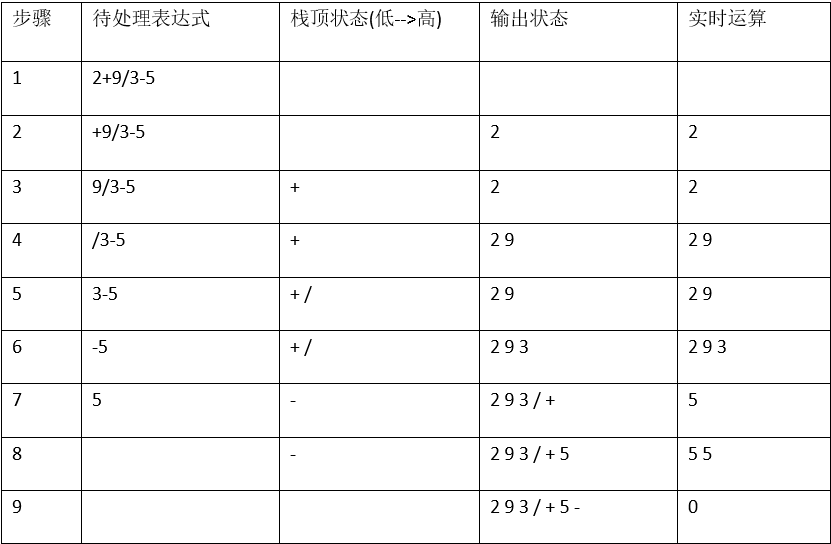带括号的运算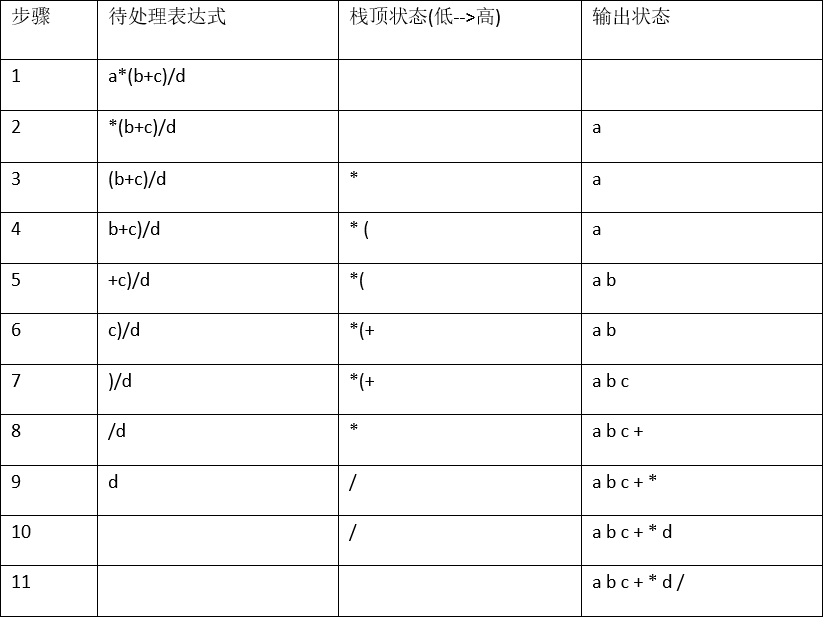选取慕课里何钦铭老师的案例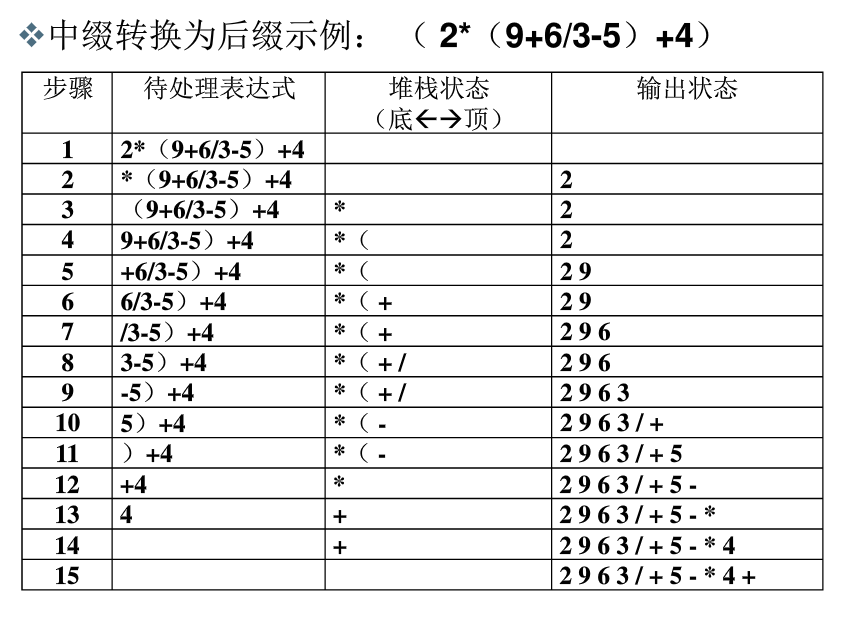后缀表达式运算步骤: (以堆栈储存) 从左到右,遇到运算符就弹出相应的运算数,运算后再把结果入栈.最终结果就是栈顶数的值. (由于该运算为线性结构,具体运算时是不需要储存输出后的运算符,一般是输出一个运算符就进行一次运算,不像图中要储存输出状态.) 注意点: 有时候’-’(负号)是单目运算符,则要修改运算数. 遇到其他运算符(如幂运算)也类似. 这篇文章只是整理中缀表达式转后缀表达式的方法和理论,目的是为了理解. 具体代码实现看我的另一篇文章(模拟表达式运算). 这部分转换对于初学者来说可能很模糊,建议去看开头链接的那个视频. 如果有什么错误或不足欢迎评论指出. 展开全文表达式运算 中缀表达式 • 后缀表达式也叫逆波兰表达式。 接下来将用后缀表达式实现复杂算术运算 系统类： midToLast():中缀表达式转后缀表达式 cal():后缀表达式计算结果 priority():计算符号优先级 GetList（）:将字符串切割存在队列里 ... 后缀表达式也叫逆波兰表达式。接下来将用后缀表达式实现复杂算术运算 系统类： midToLast():中缀表达式转后缀表达式cal():后缀表达式计算结果priority():计算符号优先级GetList（）:将字符串切割存在队列里 中缀转后缀 首先传入一个队列存储的中缀表达式； 接着创建一个新队列，一个栈。 循环读取队列的字符： 如果是数字，压入新队列。如果是左括号也直接压入新队列。如果是运算符：①如果栈为空或者栈顶为“(”或者当前运算符优先级大于栈顶运算符，入栈。 ②否则，出栈，并将出栈元素压入新队列（"("除外），一直到栈为空，或者遇到“（”或者当前元素大于栈顶元素；然后把当前元素压入栈。如果是“）”，就出栈，并将出栈元素压入新队列；一直到遇到"(",把"("出栈但并不压入队列。如果是其他符号就代表输入不符合规范。最后把循环遍历完之后，把栈里面的元素全部压入队列，队列就是后缀表达式。 后缀表达式计算结果 创建一个栈， 后缀表达式是从左往右扫描，循环遍历传入的后缀表达式。 如果字符是数字，压入栈。如果是运算符，出栈两个数字进行运算。（后出栈那个作为被减数），然后将结果入栈。循环完之后，栈中的元素就是运算结果。 正则表达式 正则表达式的好坏，决定了该程序的适用性。 . 匹配除换行符以外的任意字符。[ ] 字符类，匹配方括号中包含的任意字符。[^ ] 否定字符类。匹配方括号中不包含的任意字符*匹配前面的子表达式零次或多次+匹配前面的子表达式一次或多次? 匹配前面的子表达式零次或一次，或指明一个非贪婪限定符。{n,m} 花括号，匹配前面字符至少 n 次，但是不超过 m 次。(xyz) 字符组，按照确切的顺序匹配字符 xyz。| 分支结构，匹配符号之前的字符或后面的字符。\ 转义符，它可以还原元字符原来的含义，允许你匹配保留字符 [ ] ( ) { } . * + ? ^$ \ |^ 匹配行的开始\$ 匹配行的结束
源码
import java.util.ArrayList;
import java.util.List;
import java.util.Scanner;
import java.util.Stack;

public class demo1 {
public static String cal(List<String> base) {//后缀表达式计算结果
Stack<String> stack = new Stack<>();
for (String item : base) {
if (item.matches("[0-9]*[.]?[0-9]*")) {
stack.push(item);
}else {
float result = 0;
if (item.equals("+")) {
float tem1 = Float.parseFloat(stack.pop());
float tem2 = Float.parseFloat(stack.pop());
result = tem2 + tem1;
} else if (item.equals("-")) {
float tem1 = Float.parseFloat(stack.pop());
float tem2 = Float.parseFloat(stack.pop());
result = tem2 - tem1;
} else if (item.equals("*")) {
float tem1 = Float.parseFloat(stack.pop());
float tem2 = Float.parseFloat(stack.pop());
result = tem2 * tem1;
} else if (item.equals("/")) {
float tem1 = Float.parseFloat(stack.pop());
float tem2 = Float.parseFloat(stack.pop());
result = tem2 / tem1;
} else if (item.equals("%")) {
float tem1 = Float.parseFloat(stack.pop());
float tem2 = Float.parseFloat(stack.pop());
result = tem2 % tem1;
} else {
throw new RuntimeException("有非法字符！");
}
stack.push("" + result);
}
}
return stack.pop();
}

public static List GetList(String mes) {//将字符串分割成字符数组
String[] strings = mes.split(" ");
List<String> list = new ArrayList<String>();
for (String item : strings) {
}
return list;
}

public static int priority(String item) {//计算符号优先级
if (item.equals("+") || item.equals("-")) {
return 1;
} else {
return 2;
}
}

public static List midToLast(List<String> list) {//中缀转后缀
Stack<String> stack = new Stack<>();
List<String> newLists = new ArrayList<>();
for (String item : list) {
if (item.matches("[0-9]*[.]?[0-9]*")) {
while(item.startsWith("0")){
if(item.startsWith("0.")){
break;
}
item=item.substring(1);
}
} else if (item.equals("(")) {
stack.push(item);
} else if (item.equals("+") || item.equals("-") || item.equals("*") || item.equals("/") || item.equals("%")) {
if (stack.empty() || priority(stack.peek()) < priority(item) || stack.peek().equals("(")) {
stack.push(item);
} else {
while (!stack.empty() && !stack.peek().equals("(")) {
if (priority(item) <= priority(stack.peek())) {
}
}
stack.push(item);
}
} else if (item.equals(")")) {
while (!stack.peek().equals("(")) {
}
stack.pop();
} else {
throw new RuntimeException("有非法字符！");
}
}
while (!stack.empty()) {
}
return newLists;
}

public static void main(String[] args) {
Scanner s = new Scanner(System.in);
System.out.println("请输入中缀表达式：(每一个字符用空格隔开，目前支持的输入有数字，+-*/%，以及括号；退出请输入exit)");
String mes = s.nextLine();
while (!mes.equals("exit")) {
String result = cal(midToLast(GetList(mes)));
System.out.println("result:" + result);
System.out.println("请输入中缀表达式：(每一个字符用空格隔开，目前支持的输入有数字，+-*/%，以及括号；退出请输入exit)");
mes = s.nextLine();
}
}
}


图例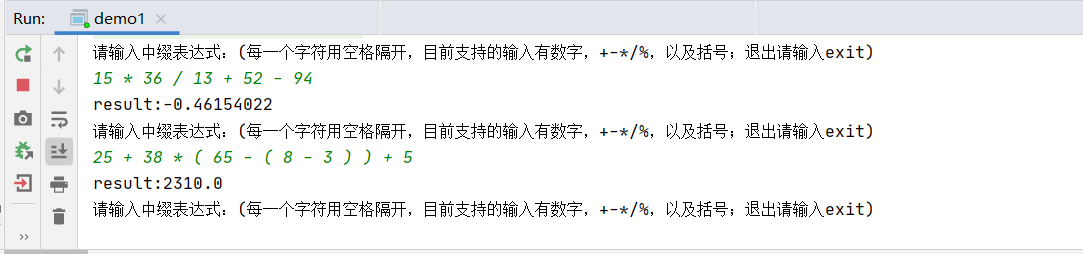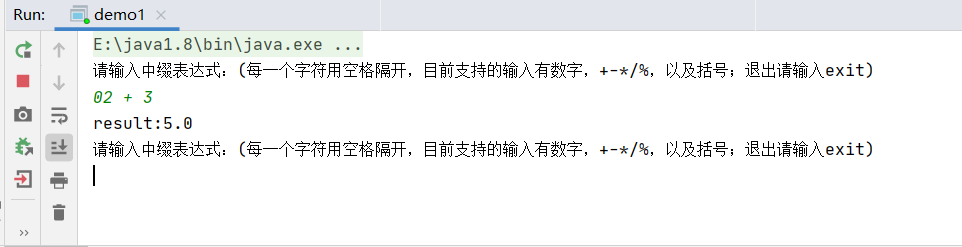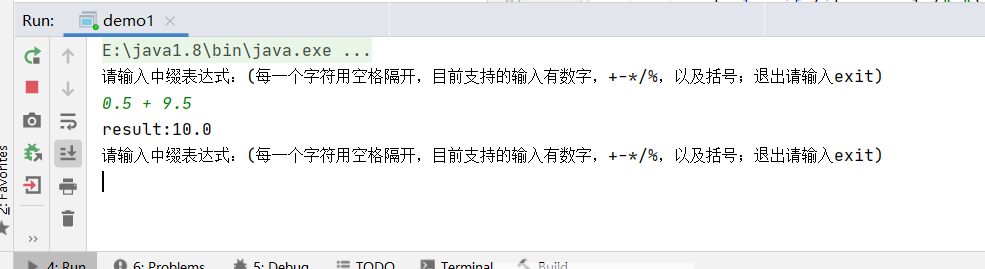温馨提示：使用栈的peek()来进行比较的时候注意不要写出pop()。
展开全文队列 java
• 中缀表达式转后缀表达式 以及 后缀表达式的计算 一、中缀表达式 转 后缀表达式 步骤： 1.准备两个空栈S1，S2 2.当遇到数字直接压入S1 3.当遇到操作符号，如果S2为空，或者当前符号的优先级大于S2栈顶操作符优先级，...
• 中缀表达式转后缀表达式 例子 中缀表达式：9+(3-1*2+7/3)*3+10/2 对应的后缀表达式： 方法一：小括号法 给每一个能加上小括号的地方都加上小括号 (9+(((3-(1*2))+(7/3))*3)+(10/2)) 然后把运算符移到小括号后面 (9...
• 后缀表达式：也称为逆波兰表达式，是将运算符写在操作数之后的表达式，例如上式的后缀表达式为：9 3 1 - 3 * + 10 2 / + 作用：对计算机而言，中缀表达式是比较复杂的结构，而逆波兰式在计算机看来却是比较简单易懂...数据结构
• 1.中缀表达式转换成后缀表达式： 首先需要注意的是：中缀转后缀的结果并不唯一。例如：（a+b+c*d）/e是一个中缀表达式，ab+cd*+e/与abcd*++e/都是其后缀表达式。 转换方式：先根据中缀表达式的各操作符的优先级，...
• // 把中缀表达式转换为后缀表达式，返回后缀表达式的长度（包括空格） void postfix(char pre[] ,char post[],int &n) {  int i = 0 ,j=0;  MyStack<char> stack;  stack.init(); // 初始化存储操作...
•数据结构
• 后缀表达式的生成树在上一篇博客 后缀表达式(1)中已经提到过，后缀表达式的优点在于它便于计算机和识别与处理。我们编程解析后缀表达式的过程，其实是生成该表达式的生成树的过程。虽然我们并不一定在代码中显式地把...二叉树 编程 编译器
• 后缀表达式： 更有利于计算机进行计算的一种表达式，不再包含括号()，也不再考虑运算符优先级，一律从左到右进行计算 举个例子： 1 2 + 二、怎么将中缀表达式转换成后缀表达式呢？ 利用栈结构来实现中缀表达式 -&...中缀表达式
• 逆波兰表达式又叫做后缀表达式。这个知识点在数据结构和编译原理这两门课程中都有介绍，下面是一些例子： 　 　正常的表达式 逆波兰表达式 　 　a+b ---> a,b,+ 　 　a+(b-c) ---> a,b,c,-,+ 　 　a+(b-c)d --...
• 2.0 什么是后缀表达式 就是把运算符放后面的表达式 例如 3 2+ 就是 3 + 2 3.0 中缀变后缀 例子3*（4+2）/2-5 1. 首先数字顺序不变： 3 4 2 2 5 2. 记住后缀表达式没有括号 3. 然后按优先级开始添运算符 .....
• 前缀表达式：不含括号的算术表达式，而且是将运算符写在前面，操作数写在后面的表达式。求法：首先从右往左扫描表达式...例子：（a + b)* (c + d) : *+ab+cd。理解：根据优先级，把数字位置不同，有那两个可以做运...中缀表达式
• ## 前缀表达式&后缀表达式

千次阅读 多人点赞 2017-08-19 17:10:50前缀表达式
•  后缀表达式中，只有操作数(1.2.3....)和操作符(+,-,*,/) --- 换句话说，就是没有所谓的小括号之类的。  操作符在两个操作数之后。  例如：平时我们习惯是2+1；写成后缀表达式，就变成了：21+ 【规则】  每...
• 中缀转后缀表达式 首先，笔者先说下中缀表达式转后缀表达式的规则 遇到字母直接输出 遇到加减乘除首先看栈中有没有运算符，没有的话直接放在栈中，有的话则比较当前元素和栈顶元素的优先级。其实这里笔者有个小技巧...数据结构
• 这就要考计算机的帮忙了，但是计算机怎么识别运算的优先级呢，这就需要前缀表达式或者后缀表达式了 先说后缀表达式 规则是从左到右遍历中缀表达式的每个数字和符号，若是数字就输出，即成为后缀表达式的一部分；若是...java 前缀表达式
•  后缀表达式：1 2 + 5 * 10 3 / + 如果将上述的中缀表达式转换为后缀表达式？ 转换原则：  1.当读取到一个数字的时候直接将其输出，不进行压栈，如果遇见操作符则不输出将其进行压栈,左括号也要
• ## 后缀表达式转中缀表达式

千次阅读 多人点赞 2017-08-17 22:39:23
在学习《数据结构与算法分析》时，第3章有一个练习题，第1问要求编写程序将中缀表达式转换成后缀表达式，这个还是容易的，因为书上已经给了详细的思路，用栈就可以了，实现方法在我另一篇博客里有写到-中缀表达式转...数据结构 算法 二叉树 递归 遍历
• ## 后缀表达式的计算

万次阅读 2016-09-11 21:57:17
1.中缀表达式转后缀表达式；2.后缀表达式的计算中缀表达式
• 给出后缀表达式，建立表达式树。后缀表达式中只有操作数和操作符，我们知道，操作数一定是表达式树的叶子结点，操作符一定是父结点。 这个过程与计算后缀表达式的值的过程极为类似。 操作数，作为叶子结点...表达式树
• 中缀表达式(Infix expression)即符合a op b的格式， 后缀表达式...举个例子，a*(b+c)/d, 转化为后缀表达式是abc+*d/, 计算机会用栈来解释执行该表达式。 如果我是计算机，对于后缀表达式输入，会做如下事情： co...
• 中缀表达式即普通的运算式子，运算符是以中缀形式处于操作数的中间(例:3 + 4)，后缀表达式就是逆波兰式(例:3 4 +)，中缀表达式转后缀表达式也是学习数据结构中的栈的时候一个典型的例子，结合上一次写到逆波兰式。...
• 中缀转后缀表达式考虑表达式 A + B * C。A B C * +是等价的后缀表达式。 我们已经注意到，操作数 A，B 和 C 保持在它们的相对位置。只有操作符改变位置。再看中缀表达式中的运算符。从左到右出现的第一个运算符为 +...算法 前缀表达式 中缀表达式 python
• 本周在写安卓计算器时用到了后缀表达式 学习之后在此奉上中缀转后缀的具体方法 拿到一个算式，建立一个临时符号栈，一个后缀表示式栈从前到后依次查看，如果遇到数字，直接放在后缀表达式栈遇到非右括号的......